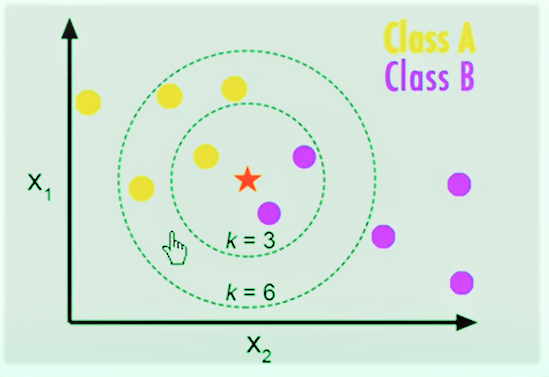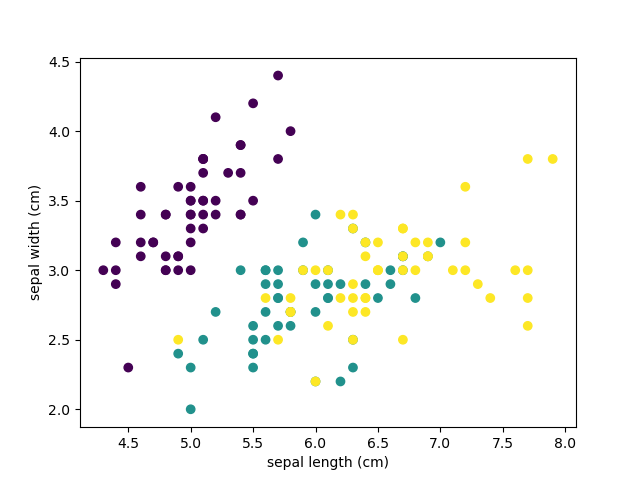# Explain KNearestNeighbours in Machine Learing in Python with examples

In this article, we will learn together the overview of the K-Nearest Neighbors (KNN) algorithm and understand the step by step implementation using KNearest Neighbors(KNN) algorithm in Python.

K-Nearest is instance-based on lazy learning method off classification. Simplest of machine learning algorithms. It provides a classification based on the distances of the labeled data w.r.t the unlabeled.

For measuring distances KNN use Euclidean distance formula i.e,Therefore, the larger k-value means the resulting curves for different complex models. Whereas, small k values ​​tend to over-fit the data and result in complex models.Note: Having the correct k-value is very important when analyzing a data-set to avoid over-fitting and under-fitting of the data-set.

Iris-Flower Classification is the best example of this algorithm.

```#Importing important libraries

import matplotlib.pyplot as plt
from sklearn.model_selection import train_test_split
import numpy as np

print(iris.keys())
print(iris.data)
features=iris.data.T
sepal_length=features
sepal_width=features
petal_length=features
petal_width=features

sepal_length_label=iris.feature_names
sepal_width_label=iris.feature_names
petal_length_label=iris.feature_names
petal_width_label=iris.feature_names
plt.scatter(sepal_length,sepal_width,c=iris.target)
plt.xlabel(sepal_length_label)
plt.ylabel(sepal_width_label)
plt.show()```

O/P Scatter plot is given below:Now you know everything about the dataset so it’s time to fit the train data by using the ‘fit()’ method.

After that, we will determine the train and test accuracy by using the ‘accuracy score()’ method. One thing can catch your attention here is that we are using k =1. You can vary the value of k and see the change in the result but the value of ‘K’ should be odd for better precision.

```from sklearn.neighbors import KNeighborsClassifier

from sklearn.model_selection import train_test_split

x_train,x_test,y_train,y_test=train_test_split(iris['data'],iris['target'],random_state=0)
knn = KNeighborsClassifier(n_neighbors=1)
knn.fit(x_train,y_train)

x_new=np.array([[5.0,2.9,1.0,0.2]])
prediction=knn.predict(x_new)

print("Predicted value is ",prediction)
print("KNN Score will be"),

print(knn.score(x_test,y_test))
```
`Output:   Predicted value is `

Predicted value  means this will fall into  class.

`KNN Score will be 0.9736842105263158`

Which means the accuracy of 97.3%.

Also read: Classification of IRIS flower